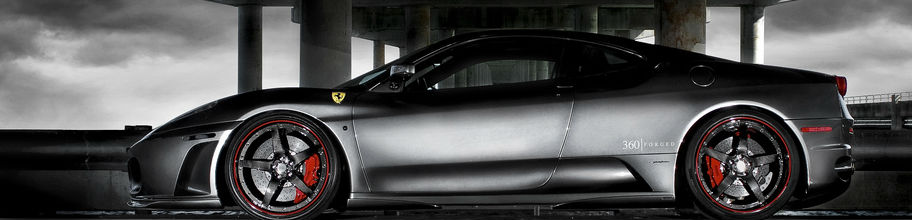## How Plus Sizing WorksTo get a tyre combination to the same as before (Factory Standard) your factory or OE (original equipment) tyres have all the details on you need to allow you to calculate the information.

Example shown – 205/50 R 16 80 Wheel Image
205mm Wheel width, 50 height in percentage (50% of 205mm), R = radius, 16 = Wheel Size, 80 = pressure & V = Speed rating (more information below) First take the tyre size, this is the width of the tyre, in this example is 205, the next number is the height of the tyre wall in a percentage, the height of the current tyre is 50% of 205 = 102.5mm. this can be worked out in this calculation, 205*50/100 = 102.5 (obviously change the width and height numbers accordingly)

Multiply the figure by 2 so you get the top and bottom wall and you will have the total height of the tyre in mm (in this case it’s the same as the width, but not always), 102.5 x 2 =205mm

Divide this by 25.4 to calculate inches this example = 8” So now we know that you have a 16” wheel (in the example above) with tyre height of 8” so a total size of 24” You can use the same calculation on the new tyres to ensure a good match

Another example 215/45 R 17 80 V
Tyre height = (45% of 215) 96.75 * 2 = 193.5 / 25.4 = 7.6” +17 =24.6” total alloy & wheel height

Now you know how to match you new wheel – tyre combination to get a similar measurement as the factory fitted wheel.